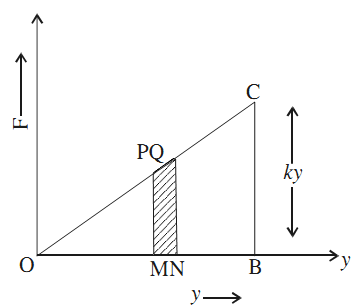# Energy of Simple Harmonic Oscillator

Simple harmonic motion can be represented by the equation

y = a sin ωt

When t changes to t + ∆t, y changes to y + ∆y.

Therefore,

y + ∆y = a sin ω(t + ∆t) = a sin (ωt + ω∆t)

= a [sin ωt cos ω∆t + cos ωt sin ω∆t]

As ∆t → 0, cos ω∆t → 1 and sin ω∆t → ω∆t. Then

y + ∆y = a sin ωt + a ω∆t cos ωt

Subtracting two equation,

∆y = ∆t ωa cos ωt

∆y/∆t = ωa cos ωt

v = ωa cost ωt

where v = ∆y/∆t is the velocity of the oscillator at time t.

Kinetic Energy

The kinetic energy of the oscillator at that instant of time is

K = ½mv2 = ½(ω2a2 cos2 ωt)

Potential Energy

When the displacement is y, the restoring force is ky, where k is the force constant. For this purpose plot a graph of restoring force ky versus the displacement y.You get a straight line graph. Take two points P and Q and drop perpendiculars PM and QN on x–axis. As points P and Q are close to each other, trapezium PQNM can be regarded as a rectangle. The area of this rectangular strip is (ky ∆y). This area equals the work done against the restoring force ky when the displacement changes by a small amount ∆y.

The area of the triangle OBC is equal to the work done in the time displacement changes from O to OB (= y) = ½ky2. This work done against the conservative force is the potential energy U of the oscillator. Thus, the potential energy of the oscillator when the displacement is y is

U = ½ky2

But ω2 = k/m

U = ½mω2y2

y = a sin ωt, s0

U = ½mω2a2sin2ωt

Total Energy

Total energy of the oscillator at any instant is given by

E = U + K

E = ½mω2a2 (sin2ωt + cos2ωt)

E = ½ma2ω2

From the graph it is evident that for y = 0, K = E and U = 0.As the displacement y from the mean position increases, the kinetic energy decreases but potential energy increases. At the mean position, the potential energy is zero but kinetic energy is maximum. At the extreme positions, the energy is wholly potential. However, the sum K + U = E is constant.